U.S. Department of Transportation
1200 New Jersey Avenue, SE
Washington, DC 20590
202-366-4000

Federal Highway Administration Research and Technology
Coordinating, Developing, and Delivering Highway Transportation Innovations

 REPORT This report is an archived publication and may contain dated technical, contact, and link information
 Publication Number:  FHWA-HRT-12-031    Date:  August 2012
 Publication Number: FHWA-HRT-12-031 Date: August 2012

# User’s Guide: Estimation of Key PCC, Base, Subbase, and Pavement Engineering Properties From Routine Tests and Physical Characteristics

PDF files can be viewed with the Acrobat® Reader®

## Resilient Modulus of Unbound Materials

The following model recommended for predicting the resilient modulus of unbound materials is based on the constitutive equation for modeling resilient modulus behavior when subjected to various stress states: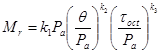Figure 100. Equation. Mr.

Where: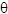=
Bulk stress =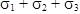.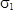= Principal stress.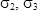= Confining pressure.
Pa = Atmospheric pressure.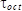= Octahedral normal stress =1/3 (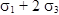).
k1, k2, k3 = regression constants that are a function of soil properties, as defined in figure 101 through figure 103 of this report.

This model can be used for various soil types, and the model attributes (k1, k2, and k3) for a given soil type remain the same regardless of stress state. Furthermore, models used to predict constitutive model attributes for a given set of soil properties are recommended to characterize resilient modulus behavior rather than developing models individually for each possible combination of expected stress states.

### Constitutive Model Parameter k1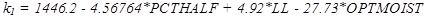Figure 101. Equation. Prediction model 18 for k1.

Model statistics for k1 are as follows:

• R2 = 0.16 percent.
• Standard error of estimate (SEE) = 237.4.
• N = 1,029.

### Constitutive Model Parameter k2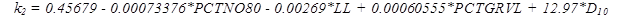Figure 102. Equation. Prediction model 19 for k2.

Model statistics for k2 are as follows:

• R2 = 0.67 percent.
• SEE = 0.0934.
• N = 1,032.

### Constitutive Model Parameter k3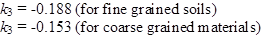Figure 103. Equation. Prediction model 20 for k3.

Where:

PCTHALF = Percent passing 1/2-inch sieve. LL = Liquid limit, percent. OPTMOIST = Optimum moisture content, percent. PCTNO80 = Percent passing No. 80 sieve. PCTGRVL = Percent gravel fraction (0.078- to 2.36-inch size). D10 = Maximum particle size of the smallest 10 percent of soil sample.

In the development of these models, a wide range of k1, k2, and k3 parameters were used, which varied by soil class. Histograms showing the distribution of k1, k2, and k3 values by soil class are shown in figure 104 through figure 106, respectively.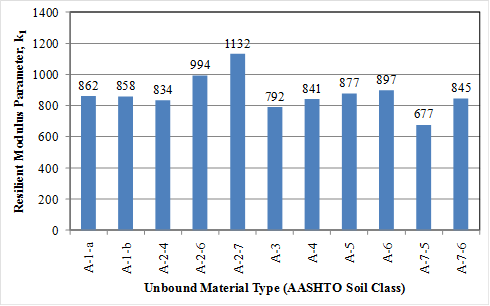Figure 104. Graph. Resilient modulus parameter k1 for unbound material types included in the model development database.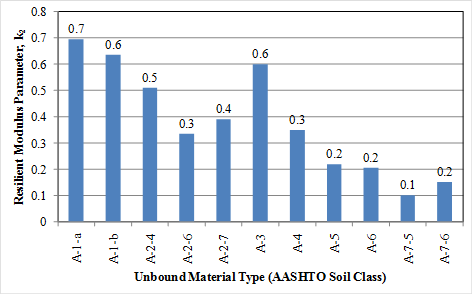Figure 105. Graph. Resilient modulus parameter k2 for unbound material types included in the model development database.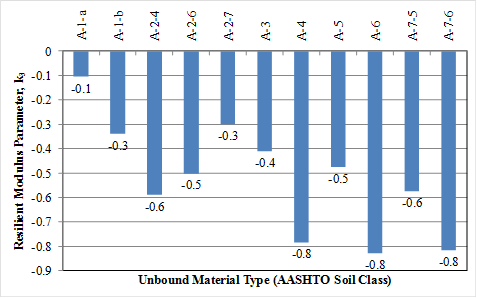Figure 106. Graph. Resilient modulus parameter k3 for unbound material types included in the model development database.

Model prediction accuracy and reasonableness were evaluated by reviewing the plot of predicted and measured resilient modulus for all individual resilient modulus test values used in model development as presented in figure 107. Figure 108 presents a plot of measured and predicted resilient modulus versus bulk stress for all fine- and coarse-grained materials included in the model development database.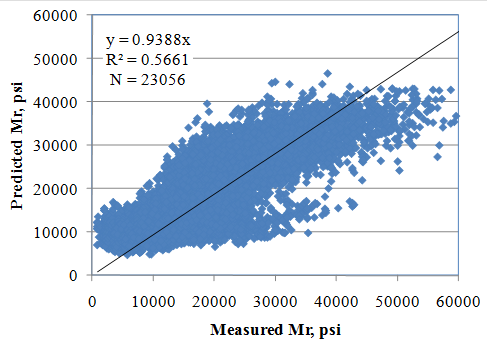Figure 107. Graph. Plot of measured versus predicted resilient modulus (using k1, k2, and k3 derived from figure 101 through figure 103).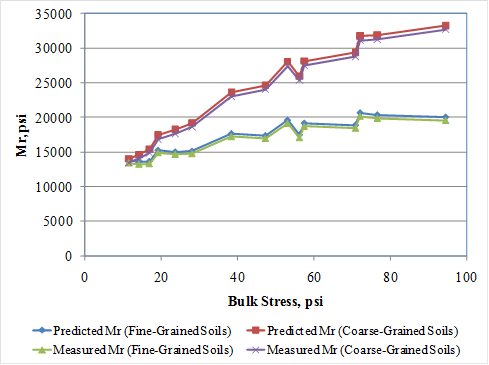Figure 108. Graph. Plot showing predicted and measured resilient modulus versus bulk stress for fine- and coarse-grained soils.

Sensitivity analysis results are presented in figure 109 through figure 115. The results of the sensitivity analysis are summarized as follows:

• Increasing bulk stress results in significantly higher level of resilient modulus for coarse-grained materials. Soil type has a significant impact on predicted resilient modulus.
• Increasing the amount of finer materials results in a decrease in resilient modulus.
• Increasing the amount of gravel results in an increase in resilient modulus.
• Increasing effective size results in an increase in resilient modulus.
• Increasing optimum moisture content results in a decreased in resilient modulus.
• Increasing liquid limit results in an increase in resilient modulus.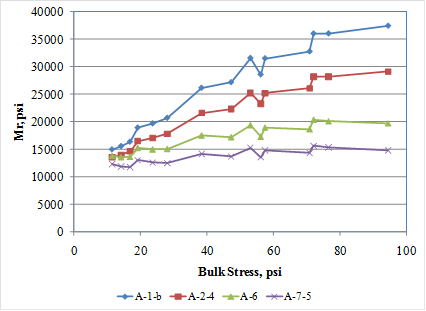Figure 109. Graph. Effect of material type (AASHTO soil class) on predicted resilient modulus.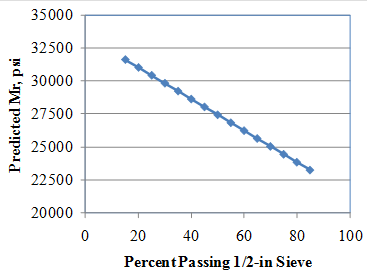Figure 110. Graph. Effect of percent passing 1/2-inch sieve on predicted resilient modulus.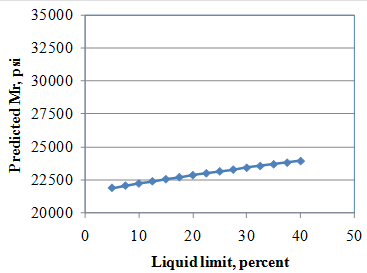Figure 111. Graph. Effect of liquid limit on predicted resilient modulus.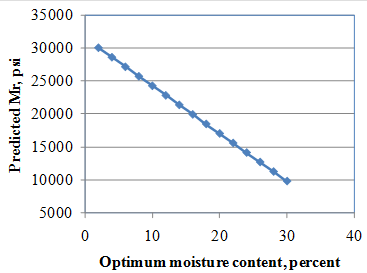Figure 112. Graph. Effect of optimum moisture content on predicted resilient modulus.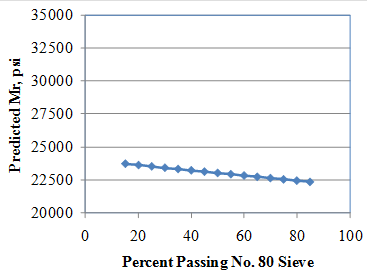Figure 113. Graph. Effect of No. 80 sieve on predicted resilient modulus.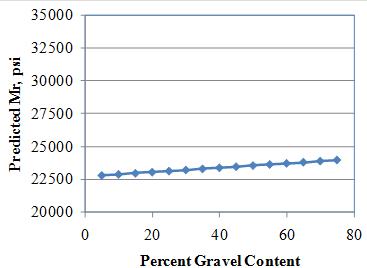Figure 114. Graph. Effect of gravel content on predicted resilient modulus.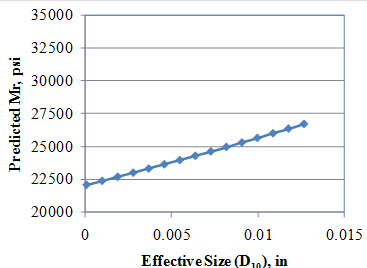Figure 115. Graph. Effect of effective size on predicted resilient modulus.

Federal Highway Administration | 1200 New Jersey Avenue, SE | Washington, DC 20590 | 202-366-4000
Turner-Fairbank Highway Research Center | 6300 Georgetown Pike | McLean, VA | 22101